Published: 20 October 2023

# Prediction of liquefaction time based on time-frequency distribution of seismic records

Hua Lu1
Yanxin Yang2
Xudong Zhan3
Ziyun Lin4
1, 2, 3, 4School of Architectural and Transportation Engineering, Guilin University of Electronic Technology, Guilin, 541000, China
Corresponding Author:
Yanxin Yang
Views 56

#### Abstract

By considering the characteristics of the time-frequency curve of ground acceleration at liquefaction sites, this research introduces the concept of a step function, defines an error function, and establishes criteria for identifying site liquefaction time. A novel method for site liquefaction time identification is proposed. One-dimensional and two-dimensional non-linear site response analysis models are established, and real liquefaction cases are studied to compare and analyze the two methods. The results demonstrate that the error function effectively identifies the moments when the frequency of the time-frequency curve of ground acceleration undergoes rapid changes, enabling the identification of site liquefaction time.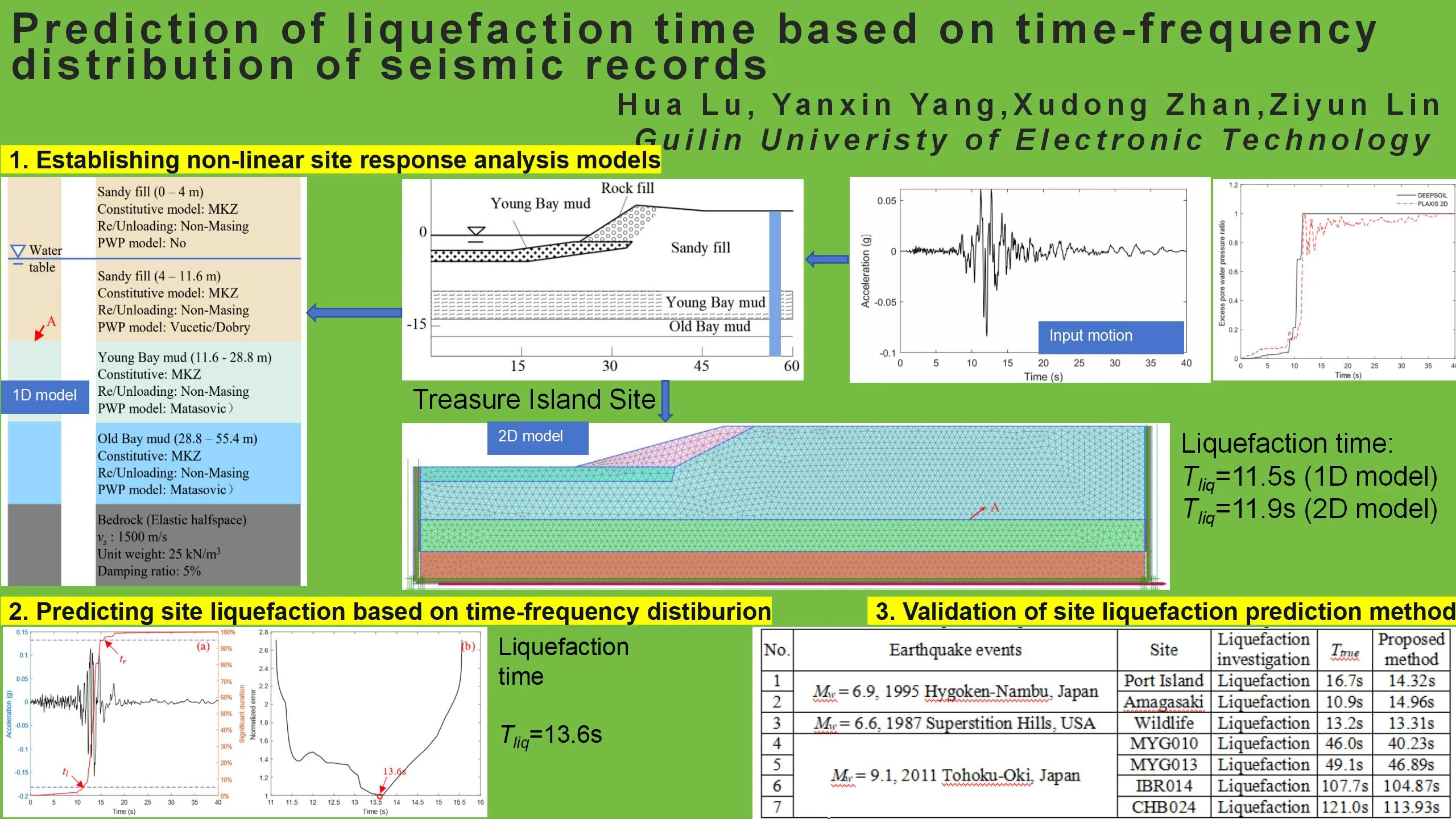#### Highlights

• Site liquefaction time depends on the site response.
• Time-frequency distribution of ground surface motion could be obtained based on HHT.
• Liquefaction time could be identified based on the phase shift or rapid change of frequency contents.

## 1. Introduction

The deformation of horizontally liquefied soil can be divided into three stages: small-magnitude seismic deformation before liquefaction, moderate-large deformation induced by seismic shaking after liquefaction, and permanent deformation after the earthquake . Therefore, identifying the liquefaction time is important for evaluating the dynamic response of the site .

Several studies have been conducted to obtain the liquefaction time of the liquefied site, Yang and Kavazajian  used FLAC 2D to construct detailed profiles of five layers and obtained the liquefaction time of the site using the PM4sand constitutive model. Özener et al.  used power spectra, combined with Stockwell spectrograms to predict the liquefaction time. Zhang et al.  developed a liquefaction time prediction model by training a CNN using time-frequency spectral. These studies [3-5] have made significant contribution to the prediction of liquefaction time, while the method used in  requires highly detailed geotechnical investigation profile, the method proposed in  is relatively complicated, and the accuracy of the method in  depends on the number of the seismic motions used in the training of convolutional neural network.

In this research, based on the distribution of seismic records in the time and frequency domains, a new method for predicting liquefaction time is proposed. The real liquefaction time of the site is taken as the benchmark for comparison, and the accuracy of the proposed method as well as non-linear site response analysis method in determining liquefaction time are analyzed.

## 2. Site response analysis

The present study focuses on the analysis of the Treasure Island site during the 1989 Loma Prieta earthquake (${M}_{w}=$ 6.9). This earthquake was characterized by various liquefaction phenomena, such as sand boiling and ground deformation . Nonlinear analysis models of the site were established using DEEPSOIL and PLAXIS 2D. The deconvolution results of the bedrock seismic waves were used as inputs for the seismic waves. The liquefaction time of the site was determined based on the changes in effective stress.

### 2.1. Site liquefaction case

The soil profile and material parameters of the site are shown in Fig. 1 and Table 1 . During the earthquake, bedrock motion was obtained near Treasure Island in Yerba Buena Island and deconvolution was performed on it. Based on the deconvolution analysis results, the horizontal ground motion records with rich energy content were selected using Arias intensity as inputs for DEEPSOIL and PLAXIS 2D analyses, as shown in Fig. 2.

Fig. 1The soil profile of Treasure Island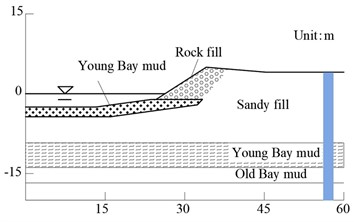Fig. 2Input earthquake records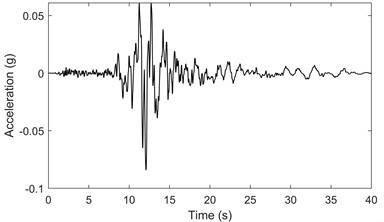Table 1Basic size and style requirements 

 Parameter Rock fill Sandy fill Young Bay mud Old Bay mud Saturation (kN/m3) 28.1 18.4 16.5 19.2 dry density (kg/m3) 23 14.11 13.1 15.23 Cohesion (kPa) 1 1 5 5 Angle of internal friction (°) 36 33 25 25 Shear wave velocity (m/s) 500 152 186 336 Poisson’s ratio 0.3 0.3 0.45 0.45

DEEPSOIL is a one-dimensional site response analysis program developed by the University of Illinois, USA. It offers three types of site response analysis methods: linear, equivalent linear and non-linear . According to reference , the sand fill material is identified as a liquefiable soil layer. To investigate the liquefaction time of the site, a soil column shown in Fig. 3 is constructed based on the blue column in Fig. 1. The stress-strain models for all layers are set as the MKZ model. The excess pore pressure model for the sand fill material below the groundwater level adopts the Vucetic/Dobry model, while the Holocene and Pleistocene Bay Mud layers use the Matasovic excess pore pressure model. The bedrock is modeled as an elastic half-space with ${V}_{s}$ of 1500 m/s, a unit weight of 25 kN/m3, and a damping ratio of 5 %. The dynamic shear modulus and damping ratio are determined based on experimental results from reference . The monitoring point for excess pore pressure is located at point A, at an elevation of –11.6 m, at the bottom of the sand fill material layer.

PLAXIS 2D is selected as the software for two-dimensional non-linear site response analysis. Based on the soil parameters of Treasure Island shown in Fig. 1 and Table 1, and referring to the research findings from reference , the following parameters are adopted. The Mohr-Coulomb model is used to generate initial stresses in the soil layers based on the available data. The UBC3D-PLM sand liquefaction model is applied to the liquefiable soil layer, while the remaining non-liquefiable soil layers are modeled using small-strain soil hardening models. The UBC3D-PLM model includes several key parameters, peak friction angle (${\phi }_{p}$), friction angle at constant volume (${\phi }_{cv}$), effective cohesion ($c$) and the normalized SPT blow count [(${N}_{1}$)60]. Among them, the value of (${N}_{1}$)60 is determined through back-analysis based on Eq. (1) . For Sandy fill, ${\phi }_{p}=$ 31.29°, ${\phi }_{cv}=$ 30.45°, $c=$ 1kPa and (${N}_{1}$)60 = 8.45:

1
${\phi }^{\mathrm{\text{'}}}=\sqrt{20\mathrm{*}\left({N}_{1}{\right)}_{60}}+20.$

Fig. 3The parameters of DEEPSOIL soil column model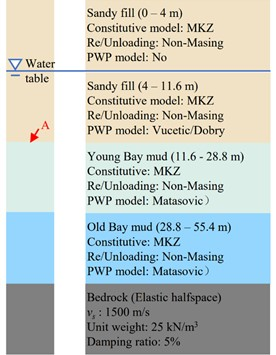To ensure that the shear strength at the lateral boundaries is not completely lost, as this could lead to excessive deformations, a Mohr-Coulomb constitutive model is applied with a 1-meter-wide drained boundary at the lateral boundaries. Additionally, to mitigate the influence of high-frequency components in the seismic motion, the Rayleigh damping method proposed in reference  is implemented. Considering the propagation theory of seismic waves in an elastic half-space, a horizontal displacement component of 0.5 meters is assigned.

Based on the above considerations, a numerical model is established with 6651 elements and 54510 nodes. The grid of model is depicted in Fig. 4.

Fig. 4Finite element analysis model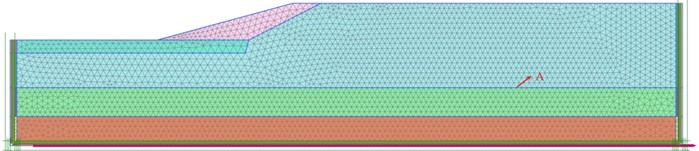The input for DEEPSOIL and PLAXIS 2D is the bedrock seismic wave shown in Fig. 2, which is used for non-linear site response analysis. The excess pore water pressure time history at location A is obtained and depicted in Fig. 5. The black solid line represents the calculation results from DEEPSOIL, while the red dashed line represents the results from PLAXIS 2D. The liquefaction time predicted by DEEPSOIL is 11.5 s, while the liquefaction time predicted by PLAXIS 2D is 11.9 s.

## 3. Prediction method of site liquefaction time

Earthquake signals are non-stationary time-domain signals, and the frequency components of the ground motion will change due to liquefaction. If the phase shift of the motions could be captured, then the corresponding liquefaction time could be obtained. Thus, this paper proposed a method for predicting the site liquefaction time as follows.

1. Select a seismic record with abundant energy in the horizontal direction based on the Arias intensity. Apply the Hilbert-Huang Transform (HHT) to process the ground acceleration time history. From the Hilbert spectrum, extract the excellent frequency curve by identifying the frequency corresponding to the maximum energy at each time instant. The specific physical interpretation of the excellent frequency is discussed in detail in reference .

2. Use the 90 % significant duration  to capture the excellent frequency time history within the corresponding time interval. Define the average frequency on the left and right beam sections at any given time as the step function, represented by ${f}_{l}$ and ${f}_{r}$.

Fig. 5The excess pore water pressure ratio time history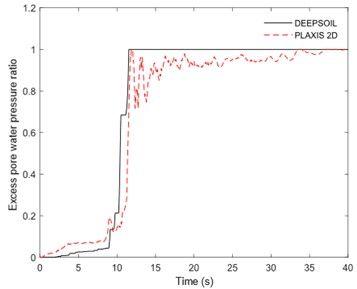3. Define the difference between the excellent frequency and the step function at any given time as the error function $E\left(t\right)$, as shown in Eq. (2). This error function effectively identifies the moments of rapid frequency change in the acceleration-time-frequency curve of the seismic record:

2
$E\left(t\right)=\frac{2{{\int }_{{t}_{l}}^{{t}_{r}}\left(f-{f}_{m}\right)}^{2}dt}{{f}_{l}+{f}_{r}},$

where, ${f}_{m}$ represents the average frequency at time $t$, $f$ represents the excellent frequency at time $t$, and ${t}_{l}$ and ${t}_{r}$ represent the start and end times, respectively, of the time interval within which the 90 % significant duration occurs.

4. Normalize the error function, and the time corresponding to $E\left(t\right)=$ 1 is considered as the estimated liquefaction time for the site.

### 3.1. Case analysis of Treasure Island

The proposed method was applied to process the ground seismic records monitored at the Treasure Island station during the 1989 Loma Prieta earthquake. The 90 % significant duration of the seismic record is shown in Fig. 6(a), from the PEER-NGA-West2 database . Additionally, the corresponding normalized error function time history is shown in Fig. 6(b).

Fig. 6a) Treasure Island acceleration time history , b) error function curve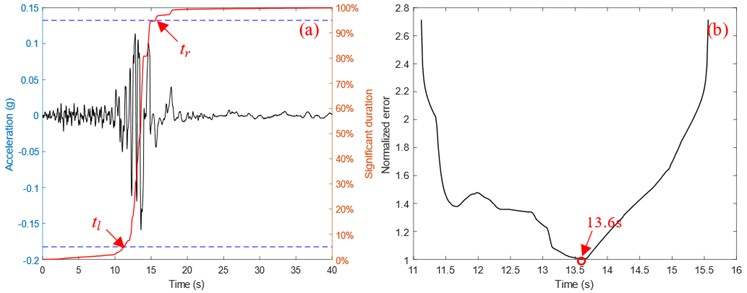The minimum value of the normalized error function corresponds to the estimated liquefaction time. From Fig. 6(b), the normalized error function occurs at approximately 13.6 s, indicating that liquefaction likely occurred at parts of the Treasure Island site at around 13.6 s.

### 3.2. Comparison of three liquefaction prediction time methods

As indicated by the published reference , it is established that the liquefaction triggering time for the Treasure Island site during the 1989 Loma Prieta earthquake event was 14.3 s. It was found that the liquefaction time for Treasure Island using the one-dimensional and two-dimensional non-linear site response analyses was 11.5 s and 11.9 s, respectively. In this paper, the proposed liquefaction time prediction method based on the prominent frequency time series resulted in a predicted liquefaction time of 13.6 s, which is very close to the true liquefaction time (with a difference of only 0.7 s). This indicates that the proposed method in this research demonstrates a high level of accuracy in predicting liquefaction time.

## 4. Validation of time-frequency site liquefaction prediction method

Based on the seven liquefaction sites with known liquefaction times from the 1995 Hyogoken-Nambu earthquake in Japan, the 1987 Superstition Hills earthquake in the United States, and the 2011 Tohoku-Oki earthquake in Japan, this paper further validates the reliability of the method for determining liquefaction times. To assess the correlation and reliability between the predicted liquefaction times and the actual liquefaction trigger times, the coefficient of determination (${R}^{2}$), as shown in Eq. (3), can be introduced. Based on a total of 7 cases in Table 2 and Treasure Island site, the calculated ${R}^{2}$ for the method is 0.99. This suggests that the proposed method exhibits a high level of reliability in predicting liquefaction time for different seismic events and sites:

3
${R}^{2}=1-\frac{\sum {\left({T}_{true}-{T}_{pre}\right)}^{2}}{\sum {\left({T}_{true}-{T}_{mean}\right)}^{2}},$

where, ${T}_{true}$ represents the true liquefaction time, ${T}_{pre}$ represents the predicted liquefaction time, and ${T}_{mean}$ represents the mean of the predicted liquefaction times.

Table 2Comparison of predicted and real values of site liquefaction time

 No. Earthquake events Site Liquefaction investigation ${T}_{true}$ Reference Proposed method 1 ${M}_{w}=$ 6.9, 1995 Hygoken-Nambu, Japan Port Island Liquefaction 16.7 s  14.32 s 2 Amagasaki Liquefaction 10.9 s  14.96 s 3 ${M}_{w}=$ 6.6, 1987 Superstition Hills, USA Wildlife Liquefaction 13.2 s  13.31 s 4 ${M}_{w}=$ 9.1, 2011 Tohoku-Oki, Japan MYG010 Liquefaction 46.0 s  40.23 s 5 MYG013 Liquefaction 49.1 s  46.89 s 6 IBR014 Liquefaction 107.7 s  104.87 s 7 CHB024 Liquefaction 121.0 s  113.93 s

## 5. Conclusions

The present study proposes a new method for predicting liquefaction initiation time based on the time-frequency characteristics of ground acceleration. The defined step function and error function in this research effectively identifies the moments of rapid frequency changes in the ground seismic records, enabling the prediction of liquefaction initiation time. A ${R}^{2}=$ 0.99 during the validation for 8 documented liquefaction sites associated with 4 seismic events indicates this method is reliable.

It should be noted that the proposed method only utilizes horizontal ground motion records. The inclusion of vertical ground motion records in the prediction of liquefaction initiation time may potentially improve the accuracy of the results.

31 July 2023
Accepted
21 August 2023
Published
20 October 2023
SUBJECTS
Seismic engineering and applications
Keywords
liquefaction site
site liquefaction time
time-frequency curve
error function
Acknowledgements

This research was supported by Guangxi Natural Science Foundation under Grant (No. 2022GXNSFBA035569) and Innovation Project of Guangxi Graduate Education under Grant (No. YCSW2023297).

Data Availability

The datasets generated during and/or analyzed during the current study are available from the corresponding author on reasonable request.

Conflict of interest

The authors declare that they have no conflict of interest.